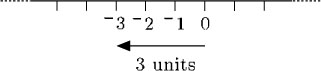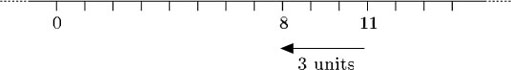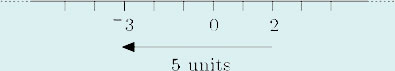Science, Maths & Technology

### Become an OU studentNumbers, units and arithmetic

Start this free course now. Just create an account and sign in. Enrol and complete the course for a free statement of participation or digital badge if available.

# 3.16 Addition of negative numbers

Now think about adding a negative number, by looking at the number line again. Suppose you start at 0. Since 0 + 3 is the same as 3, you would expect that adding 3 to 0 on the number line should take you to the point marked 3 on the number line. So, 0 + 3 = 3.

Suppose Thomas's piggy bank were empty and he added an IOU for £3 to it. The value of the piggy bank would be 3.To reach 3 from 0, move 3 units to the left. In general to add a negative number you move to the left along the number line.

Adding a negative number is the same as subtracting the corresponding positive number.

In terms of Thomas's piggy bank, a negative number is an IOU. Adding an IOU is the same as taking money out (subtracting a positive number).

## Example 25

Evaluate each of the following:

• (a) 11 + 3

• (b) 2 + 5